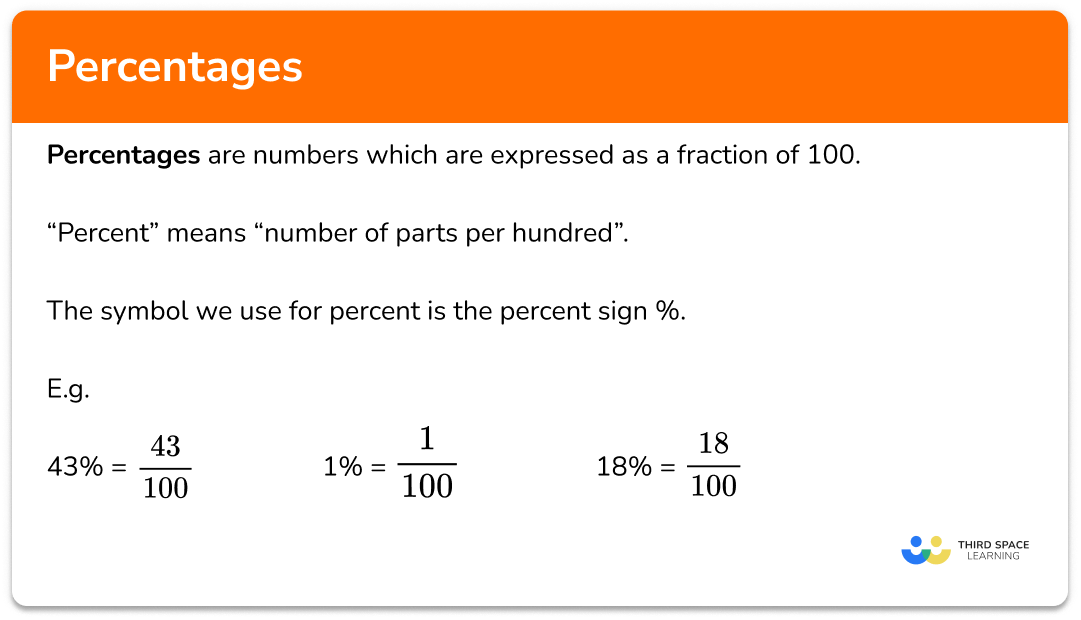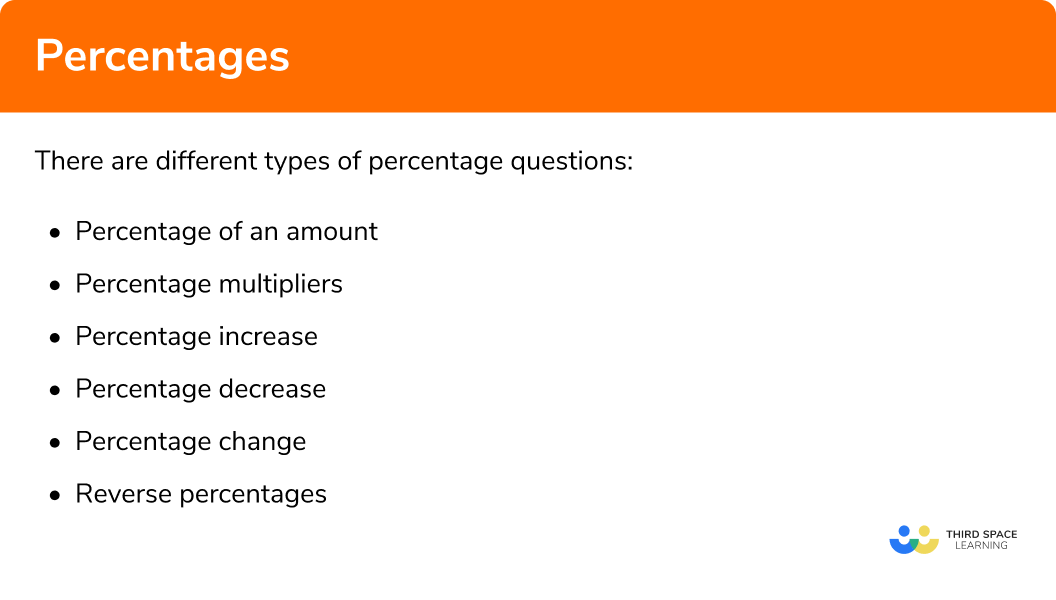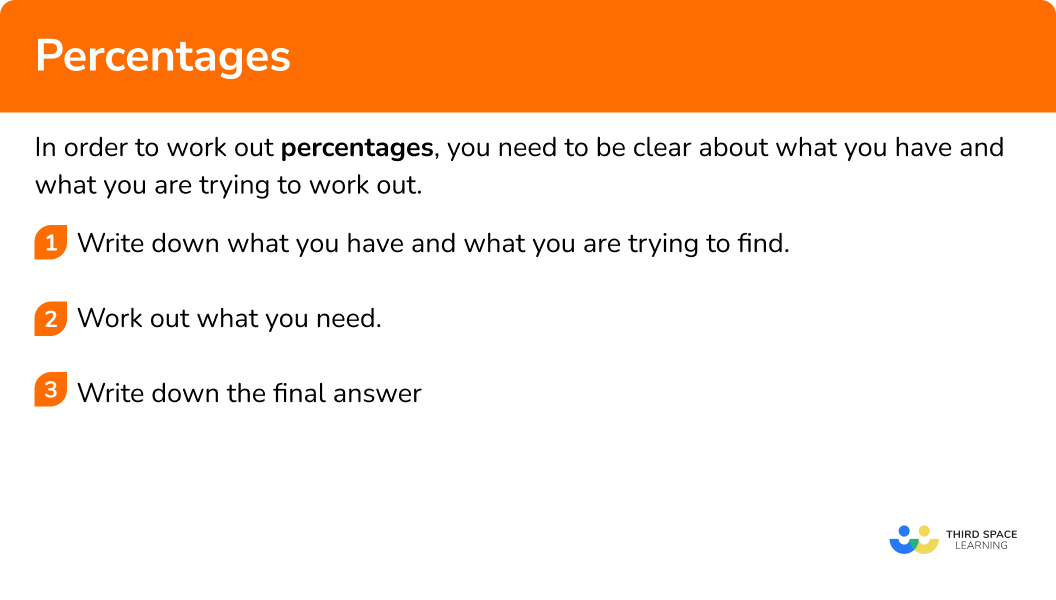# Percentages

Here is everything you need to know about percentages for GCSE maths (Edexcel, AQA and OCR). You’ll learn how to find the percentage of an amount and calculate with percentage multipliers.

You will also work out how to increase and decrease a number by a percentage, percentage change and reverse percentages.

Look out for the percentages worksheets and exam questions at the end.

## What are percentages?

A percentage is a number which is expressed as a fraction of 100

Percent means “number of parts per hundred” and the symbol we use for percent is the percent sign %.

E.g.

$43\%=\frac{43}{100}=0.43$

$1\%=\frac{1}{100}=0.01$

### What are percentages?There are different types of percentage questions.

• Percentage of an amount

This is where we are asked to find a certain percentage of an amount.

E.g. Find 25% of £32

This is the same as finding a ¼ of £32.

$32\div4=8$

Step-by-step guide: Percentage of an amount

• Percentage conversions

Percentages, fractions and decimals can all be used to represent part of a whole.

For example,

It is useful to be able to convert between percentages, fractions and decimals.

To change a percentage into a fraction, write the percentage number as the numerator of a fraction and 100 as the denominator, and then simplify the resulting fraction if possible.

E.g.

42\%= \frac{42}{100} = \frac{21}{50}

To change a fraction into a percentage, there are two methods.

First see if it is possible to write an equivalent fraction with a denominator of 100; then the numerator will be the percentage.

Eg. \frac{7}{20} = \frac{7\times5}{20\times5}=\frac{35}{100}=35\%

A second method is to carry out the division represented in the fraction and then multiply by 100.

E.g.

\frac{1}{8} = 1 \div 8 =0.125

0.125 \times 100 = 12.5

\frac{1}{8} = 12.5 \%

To change a percentage into a decimal, divide the percentage number by 100.

Eg.

73\% = 73 \div 100 = 0.73

To change a decimal into a percentage, multiply the decimal by 100.

E.g. 0.29

0.29 \times 100 = 29

29\%

• Percentage multipliers

This is where we can find a decimal number and use it as a multiplier to make calculating percentages more efficient.

E.g. Find 41% of £800

We can use 0.41 as a multiplier to find the amount needed.

$41\%=\frac{41}{100}=0.41$

$800\times0.41=328$

Step-by-step guide: Percentage multipliers

• Percentage as operator

In order to calculate a percentage of an amount, a percentage increase or a percentage decrease we can use a percentage multiplier. To do this we change the percentage that we want into a decimal, and then multiply the amount by that decimal to calculate the answer.

For example,

34% of 58.

Here we want 34% which as a decimal is 0.34

Therefore the calculation is

58 \times 0.34 = 19.72

For example,

Increase 78 by 15%

Here we want an increase of 15% which means in total we want 115%, which as a decimal is 1.15

Therefore the calculation is

78 \times 1.15 = 89.7

For example,

Decrease 45 by 20%

Here we want a decrease of 20% which means in total we want 80%, which as a decimal is 0.8

Therefore the calculation is 45 \times 0.8 = 36

• Percentage increase

This is where we are asked to increase (make bigger) a value by a certain amount.

E.g. Increase 40g by 10%.

We can find 10% and add it on.

10% of 40 is 4

$40+4=44$

Step-by-step guide: Percentage increase

• Percentage decrease

This is where we are asked to decrease (make smaller) a value by a certain amount.

E.g. Decrease 60kg by 10%.

We can find 10% and subtract it.

10% of 60 is 6

$60-6=54$

Step-by-step guide: Percentage decrease

• Percentage change

When values change, we can express this change as a percentage of the original value.

E.g. Work out the percentage change of 26kg from 25kg.

The actual change from 25 to 26 is 1.

$\frac{1}{25}=\frac{4}{100}=4\%$

Step-by-step guide: Percentage change

• Reverse percentages

This is where we are given a certain percentage of a number and we have to find the original number.

E.g. 20% of a number is 6, what is the number?

$20\%=\frac{1}{5}$

To find the original number we need to find 100% or one whole.

$5\times6=30$

Step-by-step guide: Reverse percentages

• Percentage calculations

Let’s look at different methods that can be used to perform percentage calculations.

For example,

What is 40% of 70?

Method 1: The one percent method

Find 1% first by dividing the amount by 100 and then multiply the amount by the percent you want.

\frac{70}{100}\times 40 = 28

Method 2: The decimal multiplier method

Write the percent you want as a decimal and then multiply the amount by this decimal.

40\%=0.4

70 \times 0.4=28

Method 3: Using equivalent fractions

Write the percent you want as a fraction in simplest form and then multiply the amount by this fraction.

40\% = \frac{40}{100} =\frac{4}{10} =\frac{2}{5}

70 \times \frac{2}{5} = \frac{70\times 2}{5} = \frac{140}{5} = 28

Method 4: Building up an answer from simple percentages you know

Using simple percentages you can “

build up the answer to the question.

For this question if you know 10% of 70 then you can multiply this answer by 4 to find 40%.

10\% of 70 = 7

40\% of 70 = 7 \times 4 = 28

Note, methods 1 and 2 lend themselves best to questions where you are allowed to use a calculator. Methods 3 and 4 are most helpful when calculators are not permitted.

### What are the 6 different types of percentage questions?## Equation to percentage

We could be asked to solve problems where we need to form and solve equations to find answers as percentages. We often see this when dealing with interest and depreciation calculations.

For example,

A car purchased for £36000 depreciates by x% each year. If after 3 years, the car has a value of £15187.50, what percentage does the car depreciate by annually?

If we were solving this as a depreciation question with the percentage value known and the car value unknown we would use the calculation

36000 \times \text { multiplier }^{3}=15187.50

We need to work backwards to find the multiplier. Essentially solving the equation, for ease let’s call the multiplier, x.

36000 \times x^{3}=15187.50

x^{3}=\frac{15187.50}{36000}

x^{3}=0.421875

x=\sqrt{0.421875}

x=0.75

The depreciation multiplier used for this calculation was 0.75, this tells us that the final car value of £15187.50 was 75% of its original value. 100% – 75% = 25%, so the value of the car depreciated by 25% each year.

Step-by-step guide: Percentage profit

### How to find the percentage## Percentages examples

### Example 1: percentage of an amount

Find 23% of £160.

1. Write down what you have and what you are trying to find.

100% is £160.

We need to work out 23% of £160.

2 Work out what you need.

$23\% = 20\%+3\%$

$10\% = 16, 1\% = 1.6$

$(2\times16)+(3\times1.6)=32+4.6=36.8$

3 Write down the final answer.

23% of £160 is £36.80

### Example 2: percentage multipliers

Find 39% of £4700

100% is £4700

We want to find 39% of £4700

We can write the percentage as a decimal number.

$39\%=0.39$

This gives the percentage in decimal form which is the percentage multiplier.

$4700\times0.39=1833$

39% of £4700 is £1833

### Example 3: percentage increase

Increase £200 by 30%

100% is £200

We want to find 130% of £200

$100\%+30\%=130\%$

10% is 20

$200\div10=20$

30% is 60

$3\times20=60$

So 130% is

$200 + 60 = 260$

OR

You could also use the decimal multiplier.

$100+30=130$

$130\%=\frac{130}{100}=1.30$

$200\times1.3=260$

£200 increased by 30% is £260

### Example 4: percentage decrease

Decrease 700g by 20%

100% is 700 g

We want to find 80% of 700 g

$100\%-20\%=80\%$

10% is 70

$700\div10=70$

20% is 140

$2\times70=140$

So 80% is

$700-140=560$

OR

You could use the decimal multiplier.

$100-20=80$

$80\%=\frac{80}{100}=0.80$

$700\times0.80=560$

700g decreased by 20% is 560 g.

### Example 5: percentage change

The price of a t-shirt increased from £10 to £12.  Work out the percentage change.

The original amount is £10.

We need to find the change as a percentage of the original amount.

The actual change is

$12-10=2$

The actual change written as a fraction of the original is

$\frac{2}{10}$

The actual change is the numerator (top number).
The original number is the denominator (bottom number).

Convert the fraction to an equivalent fraction where the denominator is 100

$\frac{2}{10}=\frac{20}{100}=20\%$

OR

Write the actual change as a fraction of the original amount and multiply by 100.

$\frac{2}{10}\times100=20$

The percentage change of £12 from £10 is 20%

### Example 6: reverse percentages

The price of a coat is £40 after a 20% price cut.  Find the original price.

$100\%-20\%=80\%$

£40 represents 80% of the original price.

So 80% = £40

We need the original price which is 100%.

80% is 40

We can work out 10%

$40\div8=4$

Then we can work out 100%

$5\times 10 = 50$

OR

We can make an equation using x as the original number. Use the decimal multiplier and the new number.

\begin{aligned} x\times0.80&=40\\\\ x&=40\div0.80\\\\ x&=50 \end{aligned}

The price after the cut is £40. The original price was £50

### Common misconceptions

• Money needs two digits for the pence

E.g. Find 34% of £620

$620\times0.34=210.8$

• The decimal multiplier to work out a percentage increase can be greater than 1

E.g. To increase 50km by 3% we can work 103% of 50

$50\times1.03 =51.5$

• Percentages can be greater than 100%

E.g. Calculate the percentage change from 200 to 450.

The actual change is 450-200=250

$\frac{250}{200}\times100=125$

So the percentage change from 200 to 450 is 125%

### How to work out percentage practice questions

1. Find 40\% of \pounds 300 .

\pounds 1200\pounds 120\pounds 7.50\pounds 1210\% of \pounds 300 is \pounds 30 . We can multiply this by 4 to get 40\% .

2. Calculate 12.4\% of \pounds 3000 .

\pounds 3372\pounds 37200\pounds 372\pounds 3720As a multiplier, 12.4\% is 0.124 . To get the answer we can calculate 0.124\times3000

3. Increase 600m by 30\% .

630m780m180m420m30\% of 600 is 180 . We can add this on to the original amount to find the quantity after the increase.

4. Decrease 80 kg by 5\% .

75kg95kg76kg84kg5\% of 80   is  4 . We can subtract this from the original amount to find the quantity after the decrease.

5. Calculate the percentage change from 400 kg to 600 kg .

50\%200\%100\%150\%The actual increase is given by

600  −  400 = 200

The percentage increase is then given by

\frac{200}{400}\times100=50\%

6. A book costs \pounds 4 after a price reduction of 20\% . What was the original price?

\pounds 4.20\pounds 5.00\pounds 20.00\pounds 4.25\pounds 4 represents 80\% of the original amount, which means 10\% of the original amount is \pounds 0.50 .

Multiplying by ten to get 100\% means the original price was \pounds 5 .

### Percentages GCSE exam questions

1. Work out 90\% of \pounds 70.

(2 marks)

10\% is 7 , so 90\% is 9 × 7

(1)

9\times7 = 63

(1)

2. Charlotte invests \pounds 3000 for 4 years.

She gets a simple interest rate of 2\% per year.

Work out the total interest Charlotte gets.

(3 marks)

3000\times0.02=

(1)

4\times60

(1)

= \pounds 240

(1)

3. Last year Ron paid \pounds 450 for his car insurance.

This year he has to pay \pounds 603 for his car insurance.

Work out the percentage increase in his car insurance.

(3 marks)

The change is

603-450=

(1)

\frac{153}{450}\times 100

(1)

= 34\%

(1)

## Learning checklist

You have now learned how to:

• How to work out the percentage of a number
• How to calculate percentage change
• How to work out a percentage increase or decrease

## Still stuck?

Prepare your KS4 students for maths GCSEs success with Third Space Learning. Weekly online one to one GCSE maths revision lessons delivered by expert maths tutors.

Find out more about our GCSE maths tuition programme.